# 不均衡学习的抽样方法

• 过抽样，即合理地增加少数类的样本
• 欠抽样，即合理地删减多数类样本

# 随机过抽样和欠抽样

## 随机过抽样

1. 首先在少数类 Smin$S_{min}$ 集合中随机选中一些少数类样本
2. 然后通过复制所选样本生成样本集合 E
3. 将它们添加Smin$S_{min}$ 中来扩大原始数据集从而得到新的少数类集合 Sminnew$S_{min-new}$

## 欠抽样

1. 首先我们从 Smaj$S_{maj}$ 中随机地选取一些多数类样本 E
2. 将这些样本从 Smaj$S_{maj}$ 中移除，就有 |Smajnew|=|Smaj||E|$|S_{maj-new}|=|S_{maj}|-|E|$

## 缺陷

• 对于欠抽样算法，将多数类样本删除有可能会导致分类器丢失有关多数类的重要信息
• 对于过抽样算法，虽然只是简单地将复制后的数据添加到原始数据集中，且某些样本的多个实例都是“并列的”，但这样也可能会导致分类器学习出现过拟合现象，对于同一个样本的多个复本产生多个规则条例，这就使得规则过于具体化；虽然在这种情况下，分类器的训练精度会很高，但在位置样本的分类性能就会非常不理想。

# informed 欠抽样

## EasyEnsemble 和 BalanceCascade 算法介绍

1. EasyEnsemble 核心思想是：

• 首先通过从多数类中独立随机抽取出若干子集
• 将每个子集与少数类数据联合起来训练生成多个基分类器
• 最终将这些基分类器组合形成一个集成学习系统

EasyEnsemble 算法被认为是非监督学习算法，因此它每次都独立利用可放回随机抽样机制来提取多数类样本

• 使用之前已形成的集成分类器来为下一次训练选择多类样本
• 然后再进行欠抽样

## 最近邻规则（ENN）

• 基本思想删除那些类别与其最近的三个近邻样本中的两个或两个以上的样本类别不同的样本
• 缺点：因为大多数的多数类样本的样本附近都是多数类，所以该方法所能删除的多数类样本十分有限

## 领域清理规则 (NCL)

Laur Ikkala J 等人在 ENN 的基础行提出了 领域清理规则 (neighborhod cleaning rule: NCL)。该算法的整体流程图如下所示：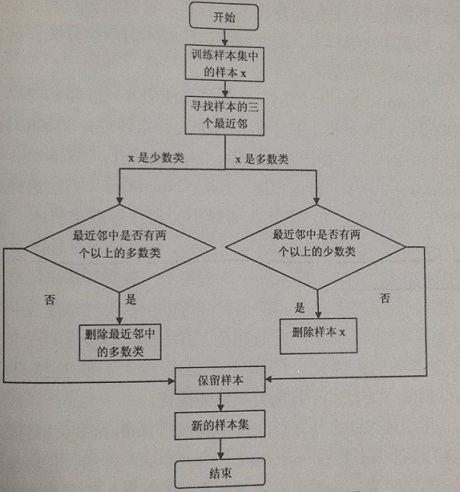• 主要思想：针对训练样本集中的每个样本找出其三个最近邻样本，若该样本是多数类样本且其三个最近邻中有两个以上是少数类样本，则删除它；反之当该样本是少数类并且其三个最近邻中有两个以上是多数类样本，则去除近邻中的多数类样本。
• 缺陷：未能考虑到在少数类样本中存在的噪声样本而且第二种方法删除的多数类样本大多属于边界样本，删除这些样本，对后续分类器的分类产生很大的不良影响。

## K-近邻（KNN）

1. NearMiss-1

2. NearMiss-2

3. NearMiss-3

4. 最远距离

Note：实验结果表明 NearMiss-2 方法的不均衡分类性能最优

# 数据生成的合成抽样方法

SMOTE 算法是利用特征空间中现存少数类样本之间的相似性来建立人工数据的。特别是，对于子集 SminS$S_{min}\subset S$ ，对于每一个样本 xiSmin$x_{i}\subset S_{min}$ 使用 K-近邻法，其中 K 是某些制定的整数。

1. 首先随机选择一个 K-近邻
2. 然后用在 [0,1] 之间的随机数乘以对应特征向量的差异
3. 最后再加上 xi$x_{i}$

xnew=xi+(x^ixi)δ$x_{new}=x_{i}+(\hat{x}_{i}-x_{i})*\delta$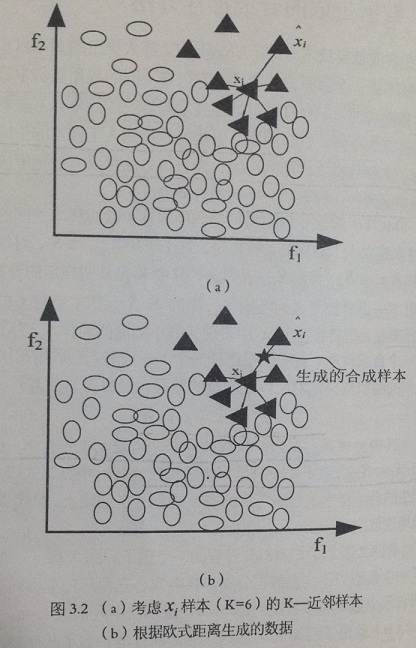、、

1. 总结

• 对于少数类的每个样本寻找其同类样本中 k 个最近邻。其中，k 通常是大于 1 的奇数
• 重复上述插值过程，使得新生成的训练数据集数据达到均衡，最后利用新的训练样本集进行训练

2. 优点

• 有助于简单打破过抽样所产生的关系
• 使得分类器的学习能力得到显著提高

3. 缺陷

• 体现在过分泛化问题和方差

# 自适应合成抽样方法

## Borderline-SMOTE 算法介绍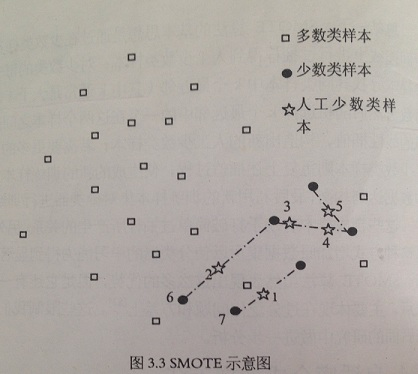## Borderline-SMOTE 算法步骤

1. 首先，对于每个 xiSmin$x_{i}\subset S_{min}$ 确定一系列最近邻样本集，称该数据集为 Si:mNN$S_{i:m-NN}$，且 Si:mNNS$S_{i:m-NN}\subset S$
2. 然后，对每个样本 xi$x_{i}$ ，判断出最近邻样本集中属于多数类样本的个数，即：|Si:mNNSmaj|$|S_{i:m-NN}\bigcap S_{maj}|$
3. 最后，选择满足下面不等式的 xi$x_{i}$m2<|Si:mNNSmaj|<m$\frac{m}{2}< |S_{i:m-NN}\bigcap S_{maj}|< m$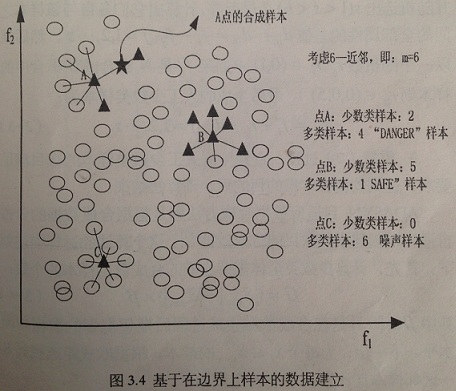## Borderline-SMOTE 流程图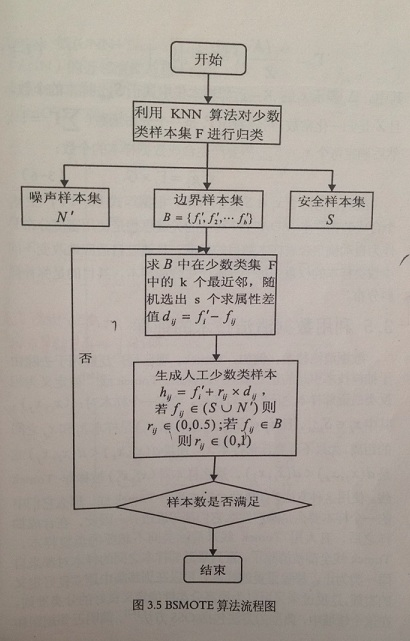（1） 步骤一
1. 计算少数类样本集 F 中每一个样本在训练样本集 T 中的 k 个最近邻
2. 然后根据这 k 个最近邻对 F 中的样本进行归类：

• 假设这 k 个最近邻都是多数类样本，则我们将该样本定义为噪声样本，将它放在 N${N}'$ 集合中
• 反之 k 个最近邻都是少数类样本则该样本是远离分类边界，将其放入 S 集合
• 最后 k 个最近邻既有多数类样本又有少数类样本，则认为是边界样本，放入 B 集合

（2）步骤二
1. 设边界样本集 B={f1,f2,...,fb}$B =\left \{ {f_{1}}',{f_{2}}',...,{f_{b}}' \right \}$，计算 B 集合中的每一个样本 fi,i=1,2,...,b${f_{i}}' , i=1,2,...,b$ 在少数类样本 F 中的 k${k}'$ 个最近邻 fij$f_{ij}$
2. 随机选出 s(1<s<b)$s (1 个最近邻
3. 计算出它们各自与该样本之间的全部属性的差值 dijdij=fifijj=1,2,...,s$d_{ij}：d_{ij}={f_{i}}'-f_{ij}，j=1,2,...,s$
4. 然后乘以一个随机数 rijrij(0,1)$r_{ij}，r_{ij} \in (0,1)$（如果 fij$f_{ij}$N${N}'$ 集合或 S集合中的样本，则 rij(0,0.5)$r_{ij} \in (0,0.5)$
5. 最后生成的人工少数类样本为：hij=fi+rijdij,j=1,2,...,s$h_{ij}={f_{i}}'+r_{ij}*d{ij}, j=1,2,...,s$

（3）步骤三

# 利用数据清洗技术的抽样

• 定义：Tomek 线被定义为相反类最近邻样本之间的一对连接
• 符号约定：给定一个样本对：(xi,xj)$(x_{i},x_{j})$，其中 xiSmin$x_{i} \in S_{min}$ ，且 xjSmaj$x_{j} \in S_{maj}$，记 d(xi,xj)$d(x_{i},x_{j})$ 是样本 xi$x_{i}$xj$x_{j}$ 之间的距离
• 公式表示：如果不存在任何样本 xk$x_{k}$，使得 d(xi,xk)<d(xi,xj)$d(x_{i},x_{k}) < d(x_{i},x_{j})$d(xj,xk)<d(xi,xj)$d(x_{j},x_{k}) < d(x_{i},x_{j})$，那么样本对 (xi,xj)$(x_{i},x_{j})$ 被称为 Tomek 线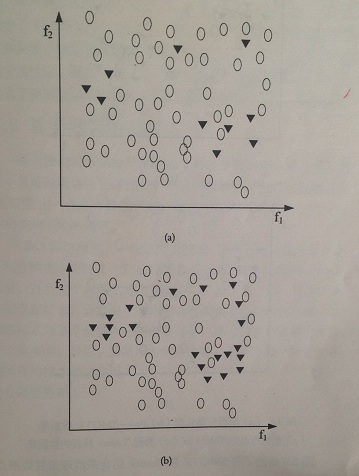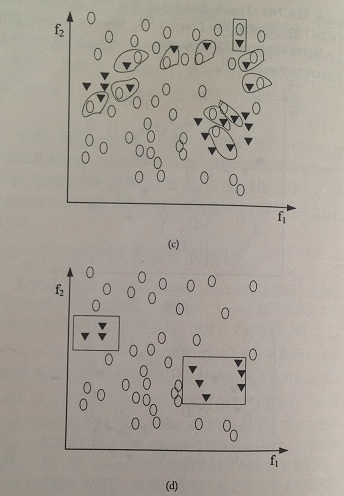(a) 原数据集分布　　　　　　　　　　　　　　　　 (c) 被识别出的 Tomek 线
(b) Post-SMOTE 数据集　　　　　　　　　　　　　(d) 移除 Tomek 线后的数据集

• 图(a) 给出了一个人工不均衡数据集的原始集分布。注意存在于少数类和多类之间的原有重复点。
• 图(b) 给出了使用 SMOTE 算法生成合成样本之后的数据集分布。可以看出，使用 SMOTE 算法是重复点增多
• 图(c) 确定了 Tomek 线，用框表示
• 图(d) 给出清理后的数据集

## 实验分析